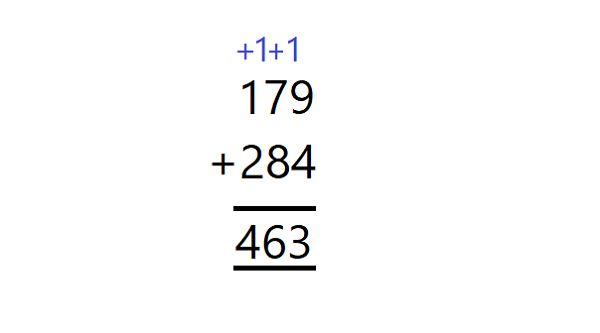# Number of carries required while adding two numbers in JavaScript

## Problem

We are required to write a JavaScript function that takes in two numbers.

Our function should count the number of carries we are required to take while adding those numbers as if we were adding them on paper.

Like in the following image while adding 179 and 284, we had used carries two times, so for these two numbers, our function should return 2.## Example

Following is the code −

Live Demo

const num1 = 179;
const num2 = 284;
const countCarries = (num1 = 1, num2 = 1) => {
let res = 0;
let carry = 0;
while(num1 + num2){
carry = +(num1 % 10 + num2 % 10 + carry > 9);
res += carry;
num1 = num1 / 10 | 0;
num2 = num2 / 10 | 0;
};
return res;
};
console.log(countCarries(num1, num2));

## Output

Following is the console output −

2

Updated on: 19-Apr-2021

209 Views